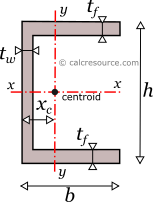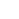Channel (U) Section Calculator

This tool calculates the properties of a U section (also called channel section or U-beam). Enter the shape dimensions h, b, tf and tw below. The calculated results will have the same units as your input. Please use consistent units for any input.

 h = b = tf = tw = Geometric properties: Area = Perimeter = xc = Properties related to x-x axis: Ix = Sx = Zx = Rgx = ADVERTISEMENT Properties related to y-y axis: Iy = Sy = Zy = xpna = Rgy = Other properties: Iz =Definitions

Geometry

The U section (also called channel) is a pretty common section shape, typically used in steel construction. However, U shaped cross sections can be formed with other materials too (e.g. concrete, aluminium, plastics, etc). The following figure illustrates the basic dimensions of a U section, as well as, the widely established naming for its components. Specifically, the U section is defined by its two flanges and the web. In this page, the two flanges are assumed identical, resulting in a symmetrical U shape.

The area A and the perimeter P of a channel cross-section, can be found with the next formulas:

The distance of the centroid from the left edge of the section , can be found using the first moments of area, of the web and the two flanges:

We reached to the last equation by dissembling the U-section in simpler components (the flanges and the web), and then finding the static moment of each one, from an axis aligned to the external edge of the web. Should you need more details about this technique, you can check our article, on finding the centroid of compound areas, here.

Moment of Inertia

The moment of inertia of a channel section can be found if the total area is divided into three, smaller ones, A, B, C, as shown in figure below. The final area, can be considered as the additive combination of A+B+C. However, since the flanges are equal, a more straightforward combination can be (A+B+C+V)-V (that is a difference between two rectangular areas). Therefore, the moment of inertia of the channel section, around centroidal x axis, is determined like this:

where h the channel height, b the width of the flanges, the thickness of the flanges and the thickness of the web. Notice that there is no need to apply the Parallel Axes Theorem, for either of the two rectangular areas (V and A+B+C+V), because their centroids lie over the examined axis x.

The moment of inertia of the channel section, around centroidal y axis, is better found with application of the Parallel Axes Theorem. The moment of inertia of the channel section, around non-centroidal y0 axis, is easy to find, if we consider the entire cross-section as an assembly of two flanges (areas B and C in figure) and one web (area A). Remember that the moment of inertia of a rectangular area, , around an axis aligned to its side is: . The following formula is then obtained:

Knowing , and centroid distance , we can calculate the moment of inertia , around centroidal y axis, using the Parallel Axes Theorem:

Why the moment of inertia is useful

The moment of inertia (second moment or area) is used in beam theory to describe the rigidity of a beam against flexure. The bending moment M applied to a cross-section is related with its moment of inertia with the following equation:

where E is the Young's modulus, a property of the material, and the curvature of the beam due to the applied load. Therefore, it can be seen from the former equation, that when a certain bending moment M is applied to a beam cross-section, the developed curvature is reversely proportional to the moment of inertia I.

Polar moment of inertia

The polar moment of inertia, describes the rigidity of a cross-section against torsional moment, likewise the planar moments of inertia described above, are related to flexural bending. The calculation of the polar moment of inertia around an axis z (perpendicular to the section), can be done with the Perpendicular Axes Theorem:

where the and are the moments of inertia around axes x and y which are mutually perpendicular with z and meet at a common origin.

The dimensions of moment of inertia are .

Elastic section modulus

The elastic section modulus of any cross section around axis x (centroidal), describes the response of the section under elastic flexural bending, around the same axis. It is defined as:

where is the moment of inertia of the section around x axis, while, Y is the distance from centroid of a given section fiber (parallel to the axis). Typically, the more distant fiber is used for this calculation, resulting in the minimum elastic modulus of the section. If a cross-section is symmetric around an axis (like the U section around x axis) and its dimension perpendicular to this axis is h, then the most distant fiber lies at a distance Y=h/2 from the axis. Therefore, the last formula becomes:

For the elastic section modulus , around the y axis, two values can be determined: one for the left fiber of the section (distance from centroid) and one for the right fibers, which are the tips of the flanges (at a distance from centroid):

where the max/min designation is based on the assumption that , which is valid for any channel section. Usually the minimum section modulus is needed only (see next paragraph why).

If a bending moment is applied on axis x, the section will respond with normal stresses, varying linearly with the distance from the neutral axis (which under elastic regime coincides to the centroidal x-x axis). Along neutral axis the stresses are zero. Absolute maximum will occur at the most distant fiber, with magnitude given by the formula:

From the last equation, the section modulus can be considered for flexural bending, a property analogous to cross-sectional A, for axial loading. For the latter, the normal stress is F/A.

The dimensions of section modulus are .

Plastic section modulus

The plastic section modulus is similar to the elastic one, but defined with the assumption of full plastic yielding of the cross section due to flexural bending. In that case the whole section is divided in two parts, one in tension and one in compression, each under uniform stress field. For materials with equal tensile and compressive yield stresses, this leads to the division of the section into two equal areas, in tension and in compression, separated by the neutral axis. This is a result of equilibrium of internal forces in the cross-section, under plastic bending. Indeed, the compressive force, realized over the entire compressive area, would be , assuming plastic conditions (i.e. the material would have yielded everywhere) and that the compressive yield stress is equal to . Similarly, the tensile force would be , using the same assumptions. Enforcing equilibrium:

The axis is called plastic neutral axis, and for non-symmetric sections, is not the same with the elastic neutral axis (which again is the centroidal one). The plastic section modulus is given by the general formula:

where the distance of the centroid of the compressive area from the plastic neutral axis and the respective distance of the centroid of the tensile area .The plastic neutral axis divides the cross section in two equal areas, provided the material features equal tensile and compressive yield strength.

Around x axis

For the case of a U-section, there is symmetry around an axis parallel to flanges. In other words, the centroidal x axis, is an axis of symmetry too. In such a case, the plastic neutral axis, that divides the whole area in two equal parts, should be also centroidal. Due to symmetry, it is and application of the last equation leads to the following formula, for the plastic section modulus, of the channel cross section, under x-x bending:

Finding centroid of the compressive area is straightforward. As shown in the following figure, the compressive area is considered equivalent to the difference, between a bigger rectangle with dimensions b and h/2, and a smaller one (blue colored). The distance is then calculated, taking into account the static moments of these subareas, like this:

where , the clear web height and the clear flange length.

Finally, the plastic modulus is found, if we substitute to the first equation:

Around y axis

U section features no symmetry, around an axis parallel to the web. If this is the case, the plastic neutral axis is not apparent by inspection alone, and needs to be determined first. The property of the plastic neutral axis to divide the whole section in two equal areas can be used. For a U-section, in particular, the following two equations are obtained for bending around y axis:

which becomes:

where is the distance of the plastic neutral axis from the external edge of the web (left edge in figure). The first equation is valid when the plastic neutral axis cuts through the two flanges, while the second one when it cuts through the web. Generally, it can't be known which equation is relevant beforehand.

Once the plastic neutral axis is determined, the calculation of the centroids of the compressive and tensile areas first and of the wanted plastic modulus afterwards, becomes straightforward. For the first case, that is when the axis crosses the two flanges, the plastic modulus can be found like this:

which can be simplified to:

where: the clear flange length.

For the second case, that is when the axis passes through the web, the plastic modulus is found with equation:

which becomes:

where: .

Radius of gyration of a cross-section, relative to an axis, is given by the formula:

where I the moment of inertia of the cross-section around the same axis and A its area. The dimensions of radius of gyration are . It describes how far from centroid the area is distributed. Small radius indicates a more compact cross-section. Circle is the shape with minimum radius of gyration, compared to any other section with the same area A. The U section however, should have considerably higher radius of gyration, particularly around the x axis, because most of the material in the section is located far from centroid.

U section formulas

In the following table, the main formulas, for the mechanical properties of the U section, are included .

Related pages

Properties of a Rectangular Tube
Properties of I/H section
Properties of unequal I/H section
Moment of Inertia of a Channel
All Cross Section tools